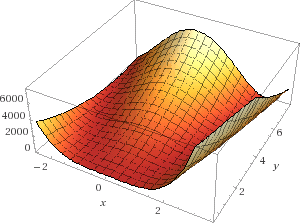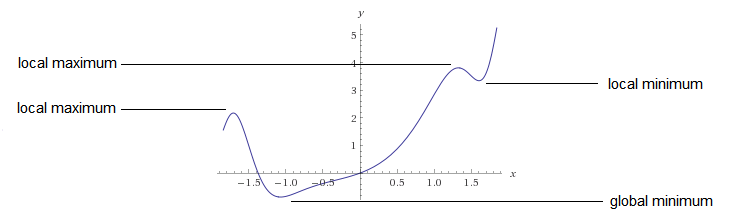# 机器学习系列(21)_SVM碎碎念part4：无约束最小化问题

## 3.从哪里着手

(w,b)w yi(wxi+b)1(i=1,,n)

“那么，如何求解这类问题？自第3部分之后大家就一直在等解决方案！！！”## 4.无约束最小化问题

f$f$fΩ$f：\Omega \rightarrow \Bbb R$x$\mathbf x^*$ 处二次连续可微。

(定理证明 ，第11页)

1. f$f$x$\mathbf x^*$ 处梯度为0:

f(x)=0

以及

2. f$f$x$\mathbf x^*$ 处的Hessian(海森矩阵)是正定的:

(zT)((2f(x))z>0,zn

此处

2f(x)=2fx212fxnx12fx1xn2fx2n

### 4.1 这些都是什么意思？

f$f$ : Ω$\Omega \rightarrow \Bbb R$x$\mathbf x^*$ 处二次连续可微。

x$\mathbf x^*$f(x)$f(\mathbf x)$ 的局部极小值点，当且仅当:

1. x$\mathbf x^*$ 用粗体书写，所以它是一个向量。这意味着f$f$ 是一个多元函数
2. 集合Ω$Ω$是函数f$f$ 的定义域。从而得出，Ω$Ω$的集合元素是向量，而且xΩ$\mathbf x^*\in\Omega$ (x$\mathbf x$ 星属于Ω$Ω$)。

f$f$x$\mathbf x^*$处梯度为0

f(x)=0

fx1(x)=0fx2(x)=0fx3(x)=0

1. Hessian(海森矩阵)是一个二阶偏导数矩阵
2. 如何判定一个矩阵是正定的

### 4.2. Hessian(海森矩阵)

Hessian是一个矩阵，也叫海森矩阵。我们可以称它为H$H$，但是这里使用2f(x)$\nabla^2f(\mathbf x)$来表示更加直白些。我们使用$\nabla$表示梯度，加上一个2$^2$来表示二阶偏导数矩阵。我们将从函数f$f$ 中计算出这些偏导数。通过f(x)$f(\mathbf x)$可以知道，函数f$f$ 的输入值是向量x$\mathbf x$ 以及 根据给定的x$\mathbf x$ 计算Hessian(海森矩阵)。

2f(x)=2fx212fxnx12fx1xn2fx2n

(注意:标量值函数的输入是一个或多个值，但返回单个值。在我们的例子中，函数f$f$ 是一个标量值函数 。)

#### 4.2.1 正定性

zT((2f(x))z>0, zn

Hessian是一个方阵，但是否是对称矩阵呢？

“如果函数f$f$ 的二阶偏导数在邻域D$D$ 中是都是连续的，那么函数f$f$ 的Hessian(海森矩阵)在邻域D$D$ 中就是一个对称矩阵”(维基百科)

• 对称矩阵A$A$是正定的

• A$A$的所有特征值都是正的

• A$A$的顺序主子式都是正的

• 存在非奇异方阵B$B$，使得 A=BTB$A=B^TB$

(来源)

• 通过计算它的特征值并检查它们是否是正数。
• 通过计算它的顺序主子式，并检查它们是否是正的。
• 通过找到一个非奇异方阵B$B$，使得A=BTB$A=B^TB$

### 4.3. 计算顺序主子式

dgfi

(dgfi)

#### 4.3.2 主子式

i=j$i = j$ 时，子式Mij$M_{ij}$ 被称为主子式

• M11=eifh$M_{11}=ei−fh$ ,
• M22=aicg$M_{22}=ai−cg$
• M33=aebd$M_{33}=ae−bd$

(nk)$\left(\begin{matrix} n \\ k \end{matrix}\right)$k$k$ 阶主子式，并把k$k$ 阶主子式表示成 Δk$\Delta k$

Δ0$\Delta _0$: 不存在，因为如果同时删除三行三列，相当于删除了整个矩阵。

Δ1$\Delta _1$:(31)=2$(3-1) = 2$，就是说我们同时删除2行和相同编号的2列得到的主子式。

i

Δ2$\Delta _2$: 就是我们之前看到的:

• M11=eifh$M_{11}=ei−fh$ ,
• M22=aicg$M_{22}=ai−cg$
• M33=aebd$M_{33}=ae−bd$

Δ3$\Delta _3$: 我们什么都不删。所以它是矩阵的行列式:

aei+bfg+cdhcegbdiafh$aei+bfg+cdh−ceg−bdi−afh$

#### 4.3.3 顺序主子式

D1=a$D_1=a$ (我们删除了最后两行的最后两列)

D2=aebd$D_2=ae−bd$ (我们删除了最后一行和最后一列)

D3=aei+bfg+cdhcegbdiafh$D_3=aei+bfg+cdh−ceg−bdi−afh$

#### 4.3.4 举例Rosenbrock   a=2 b=100

f(x,y)=fxfy

fx=2(200x3200xy+x2)fy=200(yx2)

（提示：如果你想验证你的计算结果，你可以使用数学搜索引擎Wolfram alpha)

2(200x3200xy+x2)=0(1)

200(yx2)=0(2)

400x3400xy+2x4=0(3)

200y200x2=0(4)

400xy400x3=0(5)

400x3400xy+2x4+400xy400x3=0(6)

2x4=0x=2

200y200×22=0200y800=0y=800200y=4

Hessian(海森矩阵)为:

2f(x,y)=2fx22fxy2fxy2fy22fx2=1200x2400y+22fxy=400x2fyx=400x2fy2=200

2f(x,y)=(3202800800200)

1$1$阶顺序主子式

2$2$阶顺序主子式

3202×200(800)×(800)=400$3202×200−(−800)×(−800)=400$

Hessian(海森矩阵)的所有顺序主子式都是正数。这意味着Hessian是正定的。

### 4.4 局部极小值？### 4.5 如何找到全局最小值点？

1. 找到所有局部极小值点
2. 找到所有局部极小值点中最小的一个，这就是全局最小值点。

## 5. 结论

©️2019 CSDN 皮肤主题: 编程工作室 设计师: CSDN官方博客